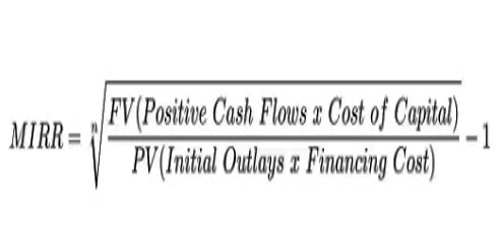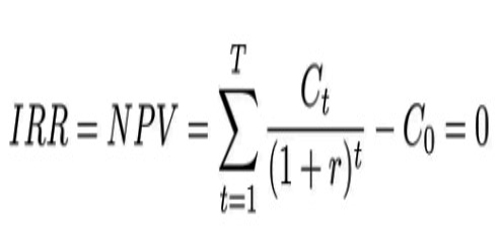MIRR –

The modified internal rate of return (commonly denoted as MIRR) is a financial measure of an investment’s attractiveness. It is used in capital budgeting to rank alternative investments of equal size. As the name implies, MIRR is a modification of the internal rate of return (IRR) and as such aims to resolve some problems with the IRR.

The MIRR is primarily used in capital budgeting to identify the viability of an investment project. For instance, if the MIRR of a project is higher than its expected return, an investment is considered to be attractive.

Conversely, it is not recommended to undertake a project if it’s MIRR is less than the expected return. In addition, the MIRR is commonly employed to compare several alternative projects that are mutually exclusive. In such a case, the project with the highest MIRR is the most attractive.Where:

FVCF(c) = the future value of positive cash flows at the cost of capital for the company

PVCF(fc) = the present value of negative cash flows at the financing cost of the company

n = number of periods

​Meanwhile, the internal rate of return (IRR) is a discount rate that makes the net present value (NPV) of all cash flows from a particular project equal to zero. Both MIRR and IRR calculations rely on the formula for NPV.

IRR –

The internal rate of return (IRR) is a statistic used in capital budgeting to evaluate the profitability of future investments. It is built on the prediction that interim cash flow is at a price parallel to the project which initiated it. The total NPV or Net Present Value of the cash flows is uniform to zero, similarly, the profit index is similar to one.

In this method, a rebate cash flow approach is observed, which examines the time and value of the funds. It’s a technique applied in budgeting that regulates the value and profit of a project. IRR evaluates the investment manifesto and compares between cut off rate and IRR.IRR is a tool used in capital budgeting that determines the cost and profitability of the project. It is used to ascertain the viability of the project and is a primary guiding factor to investors and financial institutions.

Key Differences Between IRR and MIRR –

The points given below are substantial so far as the difference between IRR and MIRR is concerned:

• Internal Rate of Return or IRR implies a method of reckoning the discount rate considering internal factors, i.e. excluding the cost of capital and inflation. On the other hand, MIRR alludes to the method of capital budgeting, which calculates the rate of return taking into account the cost of capital. It is used to rank various investments of the same size.
• The internal rate of return is an interest rate at which NPV is equal to zero. Conversely, MIRR is the rate of return at which NPV of terminal inflows is equal to the outflow, i.e. investment.
• IRR is based on the principle that interim cash flows are reinvested at the project’s IRR. Unlike, under MIRR, cash flows apart from initial cash flows are reinvested at the firm’s rate of return.
• The accuracy of MIRR is more than IRR, as MIRR measures the true rate of return.
• In some cases, the calculations of IRR may provide two solutions. This fact creates ambiguity and unnecessary confusion regarding the correct outcome. Unlike the IRR, the MIRR calculations always return a single solution.
• The common view is that the MIRR provides a more realistic picture of the return on the investment project relative to the standard IRR. The MIRR is commonly lower than the IRR.
• Another major issue with IRR occurs when a project has different periods of positive and negative cash flows. In these cases, the IRR produces more than one number, causing uncertainty and confusion. MIRR solves this issue as well.

Like the internal rate of return (IRR), the modified internal rate of return (MIRR) is not valid for ranking projects of different sizes, because a larger project with a smaller modified internal rate of return may have a higher net present value. However, there exist variants of the modified internal rate of return which can be used for such comparisons.

Information Sources: